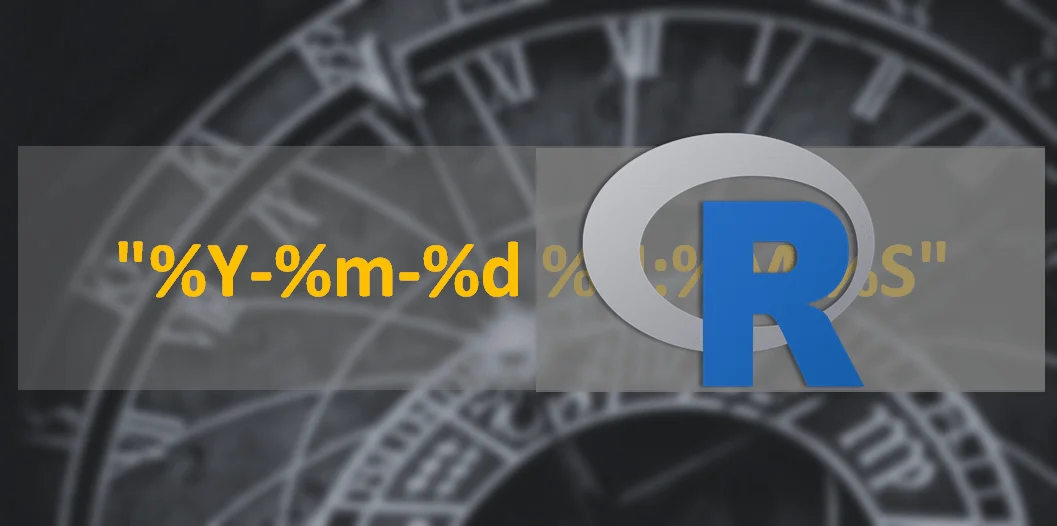# Extract date from datetime in R

Here are a couple of examples on how to extract date from datetime in R – with the results class of Date or POSIXct. If you have a large dataset, then here is my favorite solution at this moment with the function fastPOSIXct from the fasttime package that can extract date as POSIXct.

Be careful with date extraction and time zones that might look like additional time component or weird results as transformations continue. In some cases, it might lead to problems like in this example – R time shift problem after merging data.

## Extract date with class of Date form datetime in R

If you are satisfied that you are getting a result with the class of Date, then you can use the base function as.Date that is fast and simple. In this case, my dataset has only 4 million rows. When I was using it in a dataset that contains 15 million rows, it also took only seconds to extract the date.

```system.time(df\$Date <- as.Date(df\$DateTime))

#user system elapsed
#0.12 0.12 0.42```

Here are other options that might be used, but in this scenario, it is no usually reasonable.

```system.time(df\$Date <-lubridate::as_date(df\$DateTime))

#user system elapsed
#0.08 0.07 0.14

system.time(df\$Date <- anytime::anydate(df\$DateTime))

#user system elapsed
#1.52 0.18 1.76```

## Extract date with class of POSIXct from datetime in R

The best solution depends on the situation – how much data you have, and are your datetimes already formatted as POSIXct, or are they as characters. To get results in POSIXct it usually takes a longer time.

### For a small datasets

For small datasets, there are a couple of popular solutions.
Here is a good date extraction if you have a datetime column that is formatted as characters. Substr function extracts the necessary part from the left side.

```system.time(df\$Date <- as.POSIXct(substr(df\$DateTime, 0, 10), format="%Y-%m-%d", tz = "UTC"))

#user system elapsed
#38.39 4.68 47.76```

Here is another one. Both of those methods took a long time to extract that in my 4 million row dataset.

```system.time(df\$Date <-
as.POSIXct(
strftime(df\$DateTime, format = "%Y-%m-%d %H:%M:%S"),
format = "%Y-%m-%d",
tz = "UTC"
))

#user system elapsed
#20.40 1.12 22.65```

You can also use as.Date and transform the result to POSIXct, but be careful with timezones.

### Fast approach for a big datasets

When previous methods are too time-consuming, here is my favorite – fastPOSIXct form fasttime package. Your data should be in a data table format. You can do it with the function data.table from the package data table. If you are using functions like fread to import CSV files in R, then your data is already formatted like that.

```system.time(df[, Date := fasttime::fastPOSIXct(substr(DateTime, 0, 10), tz = "UTC")])

#user system elapsed
#0.77 0.11 0.97```

On my 4 million row dataset, it took only a second. Fast when compared to other solutions.

Categories R